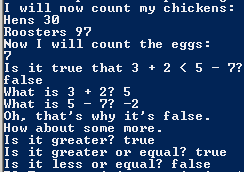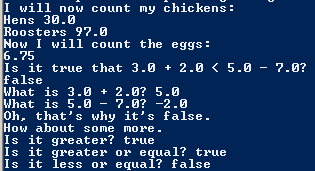# Assignemnt #11 and Numbers And Math

## Code

```
/// Name: David Shkolnikov
/// Period: 6
/// Program Name: Numbers And Math
/// File Name: NumbersAndMath.java
/// Date Finished: 9/15/2015

class NumbersAndMath {

public static void main(String[] args) {

//This line states what the person is doing.
System.out.println( "I will now count my chickens:" );

//This line is using addition to detrmine the amount of hens.
System.out.println( "Hens " + ( 25.0 + 30.0 / 6.0 ) );

//This line uses subtraction, multiplication, and division to determine the amount of roosters.
System.out.println( "Roosters " + ( 100.0 - 25.0 * 3.0 % 4.0 ) );

//This line states that the person is counting eggs now.
System.out.println( "Now I will count the eggs:" );

//This line uses lots of addition and subtraction and division to determine the amount of eggs.
System.out.println( 3.0 + 2.0 + 1.0 - 5.0 + 4.0 % 2.0 - 1.0 / 4.0 + 6.0 );

//This line uses less than symbols
System.out.println( "Is it true that 3.0 + 2.0 < 5.0 - 7.0?" );

//This line uses the same symbol
System.out.println( 3.0 + 2.0 < 5.0 - 7.0 );

//This line asks what the equation is saying.
System.out.println( "What is 3.0 + 2.0? " + ( 3.0 + 2.0 ) );

//This line does the same as the line before.
System.out.println( "What is 5.0 - 7.0? " + ( 5.0 - 7.0 ) );

//This states the reasoning for the earlier problems.
System.out.println( "Oh, that's why it's false." );

//This line makes people sad because it sais we have more work.
System.out.println( "How about some more." );

//This line asks if 5 is greater than -2.
System.out.println( "Is it greater? " + ( 5 > -2 ) );

//This line asks if 5 is greater than or equal to -2.
System.out.println( "Is it greater or equal? " + ( 5 >= -2 ) );

//This line asks the opposite of the previous line.
System.out.println( "Is it less or equal? " + ( 5 <= -2 ) );
}
}

```

### Picture of the output